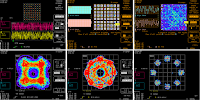## You need to test, we're here to help.

You need to test, we're here to help.

## 10 December 2015

### Using Your Oscilloscope's X-Y DisplayFigure 1: Shown are some common Lissajous patterns in an X-Y display
If you're fortunate enough to own an oscilloscope with X-Y display capabilities, you have a valuable tool at your disposal. From classic Lissajous patterns to state transition diagrams for today's quadrature communication systems, X-Y plots give us a window of the functional relationships between two waveforms.

Most users become familiar with the X-Y display by way of Lissajous patterns, where two sine waves are plotted against each other to determine their phase relationship. Figure 1 shows some commonly encountered Lissajous patterns. From them, one can gain a near-instant visual indication of how two sine waves relate in terms of phase.Figure 2: X-Y display facilitates viewing of QAM signals in a constellation pattern
If the two sine waves are in phase with a 1:1 frequency relationship, the Lissajous pattern will be linear as in the top left. With the same frequency relationship but a 45° phase difference, an oval shape results (top center). A 90° phase difference produces a circle (top right).

With a 1:2 frequency relationship and the two sine waves 90° out of phase, the Lissajous pattern assumes the bowtie shape at bottom left. Two sine waves with a 1:3 frequency relationship and 90° out of phase look like the double-bowtie at bottom center. With these general shapes in mind, an oscilloscope can provide a general validation of phase alignment between two signals.Figure 3: X-Y plots are useful in verifying phase alignment between two waveforms
An oscilloscope with X-Y display facilities also allows you to look at quadrature amplitude-modulated (QAM) signals as a constellation pattern (Figure 2).  You can perform mask tests to ensure that different constellation patterns are within a specified tolerance. In Figure 2, the pattern at the center of the top row is externally clocked and is synchronous with the device. At top right, we see a pattern that is not synchronous; the redraw areas between the constellations are clearly visible.

X-Y plots also serve to verify alignment between waveforms. At left in Figure 3 are two waveforms that are exactly in phase. Even though these are complex waveforms, we can see a linear relationship between them in the X-Y plot. At right, however, the two waveforms have slid somewhat relative to each other in time, and we get an X-Y plot that shows the misalignment.

These are some of the ways that an oscilloscope's X-Y display capabilities can help in troubleshooting the relationships between two waveforms. Let us know of other ways you've used them!